# A: Consider the following reaction:N2H5+  +  4 Fe(CN)63-  -->  N2(g)  +  5 H+  +  4 Fe(CN)64-If the starting [N2H5+] is 0.18 M and the reaction half-life is 45.0 s, what is the N2H5+ concentration after 3.00 minutes?Group of answer choices0.18 M0.045 M0.090 M0.023 M B:If the half-life for a first-order reaction is 2.54 hrs, what is the rate constant for this reaction?Group of answer choices1.76 hr-10.273 hr-1126 hr-10.693 hr-1

Question
61 views

A: Consider the following reaction:

N2H5+  +  4 Fe(CN)63-  -->  N2(g)  +  5 H+  +  4 Fe(CN)64-

If the starting [N2H5+] is 0.18 M and the reaction half-life is 45.0 s, what is the N2H5+ concentration after 3.00 minutes?

0.18 M
0.045 M
0.090 M
0.023 M

B:

If the half-life for a first-order reaction is 2.54 hrs, what is the rate constant for this reaction?

1.76 hr-1

0.273 hr-1

126 hr-1

0.693 hr-1

check_circle

Step 1

Half-life of a reaction is the time at which the initial concentration of the reactant becomes half.

The half-life of a reaction can be calculated as using the following formula :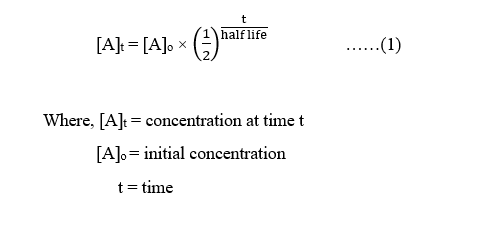Again, for a 1st order reaction, half-life is expressed as: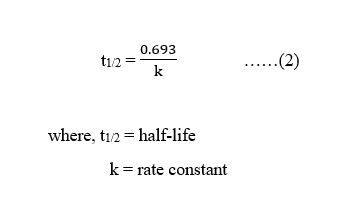Step 2

Part A :

The given reaction is :

N2H5+  +  4 Fe(CN)63-  →  N2(g)  +  5 H+  +  4 Fe(CN)64-

Initial concentration, [N2H5+]o = 0.18 M

Reaction half-life = 45.0 s

Time, t = 3.00 min = 3×60 s = 180 s

The N2H5+ concentration after 3.00 minutes, [N2H5+]t can be calculated using equation (1) as :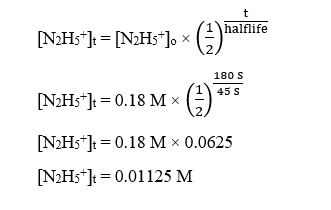The answer can be confirmed with help of the definition of half-life also.

Half-life of a reaction is the time at which the initial concentration of the reactant becomes half.

As per the definition, the concentration can be calculated as :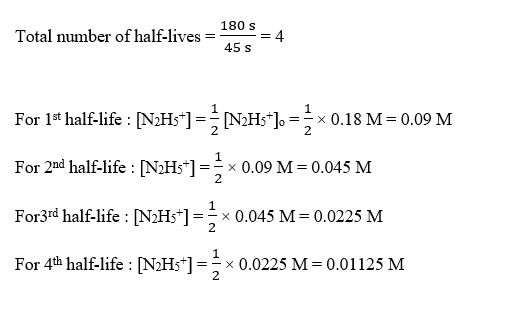The correct answer as per the values given in part A will be = 0.01125 M  (not any of the answer choices given)

Step 3

Part B :

Given,

Half-life for a first-order reaction = 2.54 hrs

The rate constant for this reaction can be calculated using equation (2) as :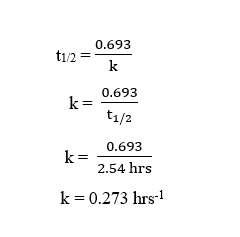...

### Want to see the full answer?

See Solution

#### Want to see this answer and more?

Solutions are written by subject experts who are available 24/7. Questions are typically answered within 1 hour.*

See Solution
*Response times may vary by subject and question.
Tagged in

### Chemistry